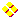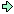World!Of
NumbersWON plate
36 |

Smallest multipliers to make a number palindromic

What is the smallest number that multiplied with 81 gives a palindrome ?
Answer : This number is 12345679
And the palindrome is a series of nine nines ! = 999999999
Note that the reciprocal of  81 is 0,0123456790123456790123...

See Sloane's A050782

Careful observation reveals that powers of  3 and/or multiples of  81
require the largest multipliers. Hence the next puzzle !
Find the smallest multipliers for the following numbers:
162, 243, 324, 405, 486, 567, 648 and 729.On [ April 27, 1997 ] I submitted this topic to sci.math but
rediscovered it only very recently [ January 2000 ] in my archive...
I got some very interesting (theoretical) replies that I like to share.
The general question was
Can every integer X be multiplied with another
integer Y to produce a palindrome P ?

Ilias Kastanas wrote after 81 * 12345679 = 999999999
That is, 10^9 = k*3^4 + 1.
It follows easily that 3^5 divides 10^27 – 1, 3^6 divides 10^81 – 1, etc.
Extra SourceNumber Theory List - Message from 17 Sep 1991Angel Garcia recalls that 1 ÷ 81 = 0.012345679 012345...
to which Glaisher adapted a famous theorem and Sierpinski derived:
1/99^2 = 0.00 01 02 03 ... 97 99 00 01 ...
and similarly (Lahoz)
1/999^2 = 0.000 001 002 003 .......
Angel Garcia thinks that it is a matter of finding a LARGE enough
integer to palindromize 81.David G Radcliffe generalized that every positive integer is a factor
of a palindrome, unless it is a multiple of 10.

 If X is not divisible by 2 or 5, then 10^p = 1 mod X, where p = phi(X) is the number of integers between 1 and X which have no common factor with X. In this case, put Y = (10^p – 1) / X. Then X * Y = 10^p – 1 is a palindrome. If X is even, then write X = 2^n * W, where W is odd. Construct a palindrome Q = 10^n * R + 2^n, where R is the digit reversal of 2^n. Let p = phi(W). Then 10^(2pn) = 1 mod W, so S := 1 + 10^(2pn) + 10^(4pn) + ... + 10^(2(W–1)pn) = 0 mod W. Let P := Q*S. Then P is a palindrome which is divisible by X. A similar construction can be used when X is a multiple of 5. Example: if X = 81, then p = 54, and Y = (10^54 – 1)/81. 81 * 12345679012345679012345679012345679012345679012345679 = 999999999999999999999999999999999999999999999999999999 Example: If X = 112, then X = 2^4 * 7, Q = 610016, and p = 6. Then P = 610016 0..0 610016 0..0 610016 0..0 610016 0..0 610016 0..0 610016 The 610016's are placed to ensure that P is divisible by 7, while 610016 itself is divisible by 16. Therefore P is a multiple of 112.We might also note that phi(3^k) is always even, so we
have phi(3^k) = 2m and (10^2m – 1) = (10^m – 1)(10^m + 1).
Clearly the second term in the factorization is not divisible
by 3, so 3^k must divide 10^m – 1. Similarly, m is always
divisible by 3, and we can show that 10^(m/3) – 1 is divisible
by 3^k, as can be seen from the fact that

$$10^{3^k}-1 = (10-1)*\prod_{j=0}^{k-1}\ [\ x^{2*3^j}+x^{3^j}+1\ ]$$ The term (10 – 1) is divisible by 2 powers of 3, and each term
inside the product is divisible by exactly 1 power of 3.
This shows that 3^k not only divides 10^phi(3^k) – 1 (by
Euler's theorem), it also divides 10^(phi(3^k)/6) – 1.
For example, we have

81 * 12345679 = 10^9 – 1 = 999999999
243 * 4115226337448559670781893 = 10^27 – 1
and so on.David M. Einstein remarks after reading
243 divides 10^162–1
739 divides 10^486–1
that these are almost certainly not the smallest solutions, but they show
that 3^n can always be made palindromic. In general if k is not divisible
by 2 or 5 then k divides 10^n–1 where n is the number of positive integers less
than k that do not share a common factor with k (look at an elementary number
theory book for a description of the totient function).

and when Hauke Reddmann asked if 12345679 is the lowest factor
which turns 81 palindromic (81 * 12345679 = 999999999), David replied :

12345679 is the smallest multiplier that makes 81 palindromic. This is
an interesting side effect of the fact that 10^n = n*9 + 1 mod 81.
An n+1 digit palindrome divisible by 81 can be written as
a_0 * (10^n+1) + a_1(10^(n–1)+10) + ... = 0 mod 81
with 0 ⩽ a_i ⩽ 9 and a_0 ≠ 0
this turns out to be
(9n+2)(a_0+...+a_{(n–1)/2}) = 0 mod 81 if n is odd
or
((9n+2)/2)(2 a_0 + ... + 2 a_{(n–2)/2} + a_{n/2}) = 0 mod 81

Since 9n+2 and ((9n+2)/2) are units mod 81, the sums must = 0 mod 81. It is
easy to see that the first time that this happens is at 999999999.Kurt Foster distinguishes four cases in the Palindrome proof :

 Case (1) X is itself a palindrome -- in this case, P = X works. Case (2) X isn't a palindrome, and is relatively prime to 10. In this case, there is a least positive integer N (a divisor of phi(X)) such that X divides 10^N – 1; P = 10^N – 1 works, though it may not be the smallest palindrome divisible by X. Case (3) X = x * 2^m, where gcd(x, 10) = 1. Here, we first find a palindrome p divisible by 2^m. If 2^m isn't a palindrome, then one such p may be constructed as follows: pad 2^m out to m places by padding "on the left" with zeros, then prepend this with the digits of 2^m IN REVERSE ORDER. Anyhow, if p has b digits, then Q = (10^(b*N) – 1)/(10^b – 1) will be divisible by x for a suitable N = N(x, b); and P = p * Q (the b-digit palindrome p repeated N times) will be a palindrome divisible by X. This may not be the smallest P that works, though. Case (4) X = x * 5^m, x as in Case (2). Similar to Case (3).Many other people like Keith Lynch, Lynn Johannesen or Keith Ramsay
emailed that 999999999 is definitely the smallest palindrome divisible by 81
and with quotient 12345679.
I quote also Fred W. Helenius : "... It is indeed remarkable that one must go so far."Then came Barry Wolk with yet another line of approach.

We were asked for a palindrome divisible by 81, and for one divisible by 3^5,
one by 3^6, etc. David Radcliffe gave a mathematical answer, based on
the result that, whenever n is not divisible by 2 or 5, 10^phi(n) – 1 is divisible by n.
Here is a more elementary answer :

9 is divisible by 9, and 111 is divisible by 3, so their product
is divisible by 9*3 = 27. This product is the palindrome 999.

999 is divisible by 27, and 1001001 is divisible by 3, so their product
is divisible by 27*3 = 81. This product is the palindrome 999999999.

999999999 is divisible by 81, and 1000000001000000001 is divisible by 3,
so their product is divisible by 81*3 = 3^5. This product is the palindrome 10^27 – 1.

This pattern continues, and it gives a proof by induction that 10^n – 1
is divisible by 9n whenever n is a power of 3.

If you want palindromes that aren't of the form 10^n – 1, you can use
a similar procedure to show that, for example:

90909 is divisible by 27
99909990999 is divisible by 81
99999999909999999990999999999 is divisible by 243
etc.

It is easy to show that 999999999 is the smallest palindrome divisible by 81.
Keith Lynch already posted that result, by a computer search. Try proving this
without an exhaustive computer search (although hand calculators are allowed).
Is there a palindrome smaller than 10^27 – 1 that is divisible by 243 ?
Barry finally asks as an extra puzzle.Fred W. Helenius solved Barry Wolk's puzzle !

Yes, 243 divides 29799999792.

And, before you ask (these are all minimal):

729 divides 39789998793,
2187 divides 39989598993, and
6561 divides 68899199886.

The last one is the amazing one: not only is it divisible by 3^8;
it is divisible by 3^12 = 531441.
For a more challenging puzzle, find the smallest palindrome divisible by 8181.
Solution found by Patrick De Geest on [ March 10, 2001 ]Lynn Johannesen investigated the case 2*81 or 162.

Now try 162 (=2*81). Exhaustive search demonstrates that there's
no answer where the product is less than 2^32. On the other hand, the number
composed of 81 2's works, by an argument similar to Keith Lynch's
for 999999999/81. Is there anything smaller ?

Fred W. Helenius replied positively to Lynn's question.
162 * 172839506 = 27999999972 (=28*999999999).From here on the discussion extinguished...
Allow me to recapitulate the results in the following table.

Integer XSmallest MultiplierPalindrome
81 or 3412345679999999999
16217283950627999999972
243 or 3512263374429799999792
3248641975327999999972
40513580246954999999945
4866131687229799999792
5674938271627999999972
6484598765429799999792
729 or 365458161739789998793
8916172839554999999945
9723065843629799999792
10532744539428899999882
11342469135827999999972
.........
2187 or 371828513939989598993
6561 or 381050132668899199886
818111734506782789399599999998999999959
19683 or 39350044268899199886
59049 or 310116681468899199886
81818111122233344455566677788990999999999999999999999999909

It looks like the concatenations of 81
are the most difficult cases to solve...

Strings
of 81
Multiplier Infinite Pattern
by Patrick De Geest
Strings
of 9
(81)1 or 811(2)13(4)15(6)17(8)09 0(1)12(3)14(5)16(7)19
12345679012345679
18
(81)2 or 81811(2)33(4)35(6)37(8)29 0(1)32(3)34(5)36(7)39
122234445666788901112333455567779
36
(81)3 or 8181811(2)53(4)55(6)57(8)49 0(1)52(3)54(5)56(7)59
1222223444445666667888890111112333334555556777779
54
(81)k1(2)2k–13(4)2k–15(6)2k–17(8)2k–29 0(1)2k–12(3)2k–14(5)2k–16(7)2k–19k * 18

Strings
of 81
Smallest Possible MultiplierPalindrome
8112345679999999999
81811173450678278939 - [ March 10, 2001 ]9599999998999999959
818181111222333444555666777889
by Daniel Fischer [ June 19, 2008 ]
90999999999999999999999999909
81818181
11117333444506667778277788893889
by Daniel Fischer [ June 20, 2008 ]
909599999999999999989999999999999995909

It looks like the sequences 89...91 generates
some large minimal multipliers, too (by Chen Xinyao with 891 & 8991,
(Daniel Fischer with 89991, 899991 and larger) :

Sequences
of 89...91
Smallest Possible MultiplierPalindrome
891
6172839554999999945
8991
111222333444555666777889999999999999999999999999999
89991
116788345501105666111050989999998999999890501
899991
1111122222333334444455555666667777788889999999999999999999999999999999999999999999999
8999991
116772327883438994550105661110509498999999999999999999989490501
89999991
11111112222222333333344444445555555666666677777778888889999999999999999999999999999999999999999999999999999999999999999
(22 minutes)Continued at WONplate 96 and WONplate 223A000036Prime Curios!Prime PuzzleWikipedia 36Le Nombre 36Numberland 36[TOP OF PAGE]

Patrick De Geest - Belgium- Short Bio - Some Pictures